# 浅谈推荐系统

## 1. 常用评测指标

1. 用户满意度：用户是推荐系统中非常重要的参与者,他们的满意度也直接决定了推荐系统的好坏.但是用户满意度这个指标无法离线计算,只能通过用户调查或者在线实验获得，一般都是线上行为统计，包括购买率（CVR），点击率（CTR），用户停留时间，转化率等等。

2. 预测准确度：预测准确度是用来度量用户的实际行为与推荐系统预测结果的准确度,该指标是最重要的离线评价指标,因为可以通过离线计算得到.下面是预测准确度最常用的两个指标。

1. 评分预测：预测用户对物品的评分行为，这种评分模型的预测准确度一般使用均方根误差（RMSE）和平均绝对误差（MAE）。其中用户$u$, 物品$i$， 实际 评分$r_{ui}$， 预测评分$\hat{r_{ui}}$。则对应的RMSE和MAE为：

$RMSE=\sqrt{\frac{\sum _{u,i\in T}\left ( r_{ui}- \hat{r_{ui}}\right )^{2}}{\left | T \right |}}$
$MAE=\frac{\sum _{u,i\in T}\left | r_{ui}- \hat{r_{ui}} \right |}{\left | T \right |}$
2. TopN推荐：推荐系统在给用户推荐物品的时候,往往会给用户一个列表的推荐物品,这种场景下的推荐是TopN推荐,该推荐方式最常用的预测准确率指标一般是精确率(precision)和召回率(recall)。其中$R(u)$为推荐列表，$T(u)$为实际行为列表。

$Precision=\frac{\sum _{u,i\in T}\left | R(u)\cap T(u))) \right |}{\sum _{u,i\in T}\left | R(u)) \right |}$
$Recall=\frac{\sum _{u,i\in T}\left | R(u)\cap T(u))) \right |}{\sum _{u,i\in T}\left | T(u)) \right |}$

有时候为了更加全面的评估TopN推荐,通常会选取不同的推荐列表长度计算多组精确率与召回率然后分别绘制出精确率曲线和召回率曲线,需要注意的是这里并不是PR曲线,感兴趣的可以了解一下PR曲线相关的知识。

3. 覆盖率：描述一个推荐系统对物品长尾的发觉能力，一个简单的定义可以是:推荐系统所有推荐出来的商品集合数占总物品集合数的比例. 但是商品数太集中在几个商品集合的话也不行，可以通过研究物品在推荐列表中出现的次数分布来描述推荐系统挖掘长尾的能力,如果这个分布比较平缓说明推荐系统的覆盖率比较高,而如果分布比较陡说明推荐系统的覆盖率比较低.下面分别使用信息熵基尼系数来定义覆盖率.

1. 信息熵定义覆盖率: 其中$p(i)$是物品$i$的流行度除以所有物品流行度之和

$H = -\sum_{i=1}^n p(i) logp(i)$
2. 基尼系数定义覆盖率: 其中$i_j$是按照物品流行度p从小到大排序的物品列表中第$j$个物品

$G=\frac{1}{n-1} \sum_{j=1}^{n}(2j-n-1)p(i_{j})$
4. 多样性: 度量推荐列表中所有物品之间的不相似性。可通过不同的相似性函数度量推荐列表中的商品的相似性，比如商品基于内容的相似,基于协同过滤的相似,这样就可以得到不同角度的多样性。令函数$s(i,j)$为物品$i$和物品$j$的相似性,那么用户推荐列表的多样性可以定义为:

$Diversity(R(u))=1-\frac{\sum_{i,j \in R(u)}s(i,j)}{\frac{1}{2}|R(u)|(|R(u)|-1)}$

推荐系统整体的多样性可以定义为所有用户推荐列表多样性的平均值:

$Diversity = \frac{1}{U} \sum_{u\in U}Diversity(R(u))$
5. 新颖性:满足推荐的新颖性最简单的方法就是给用户推荐他们之前没有看过的物品,但是每个用户没见过的物品数量是非常庞大的,所以一般会计算推荐物品的平均流行度,流行度越低的物品越有可能让用户觉得新颖,因此,如果推荐结果中的物品平均热门程度比较低说明推荐的结果就可能比较新颖.

6. AUC曲线:AUC（Area Under Curve），ROC曲线下与坐标轴围成的面积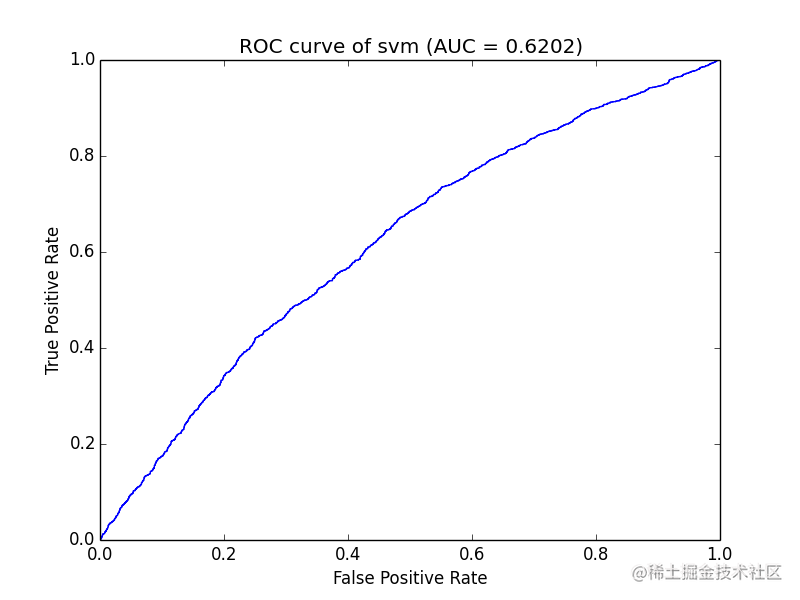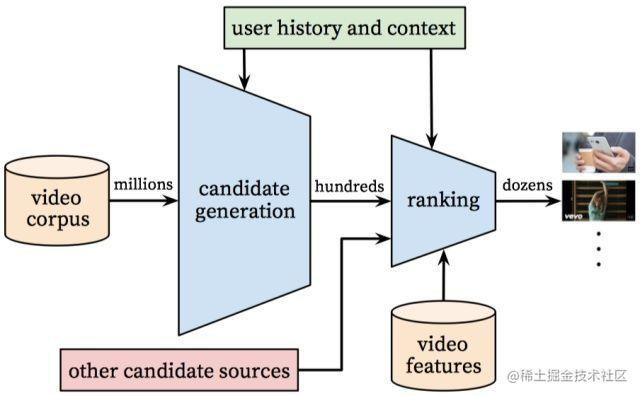## 2. 召回

### 2.1 召回层在推荐系统中的位置和作用

• 召回阶段往往会利用少量的特征简单的模型对大规模的数据集进行快速筛选
• 排序层一般会使用更多的特征和更加复杂的模型进行精准排序

• **召回层：**待计算的候选集合大、计算速度快、模型简单、特征较少，尽量让用户感兴趣的物品在这个阶段能够被快速召回，即保证相关物品的召回率
• **排序层：**首要目标是得到精准的排序结果。需要处理的物品数量少，可以利用较多的特征，使用比较复杂的模型。

### 2.2 多路召回策略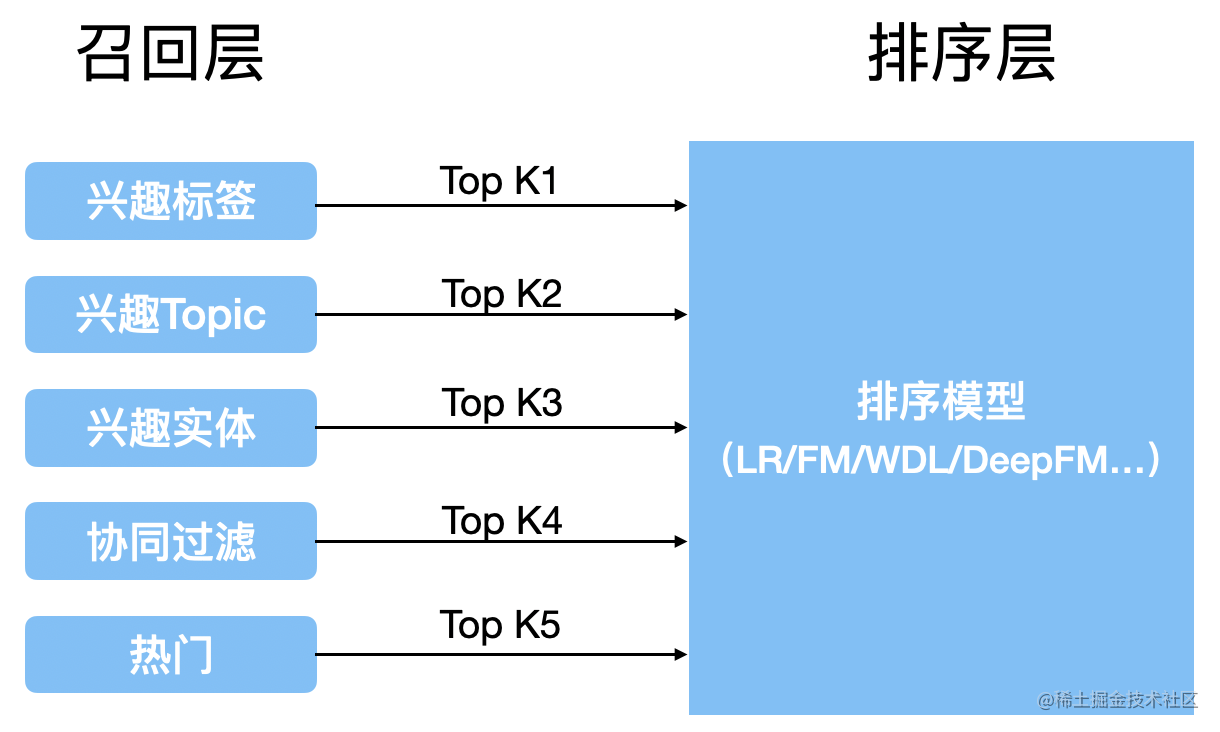### 2.3 Embedding召回

Embedding其实是一种思想，主要目的是将稀疏的向量(如one-hot编码)表示转换成稠密的向量。

• text embedding: 目前使用最多的embedding技术，对于文本特征可以直接使用该技术，对于非文本的id类特征，可以先将其转化成id序列再使用text embedding的技术获取id的embedding再做召回
• 静态向量：word2vec, fasttext, glove.
• 动态向量：ELMO, GPT, BERT
• image embedding: 根据图像或视频特征，用预训练model（提取图像或视频向量特征），然后用于召回。
• graph embedding: 这个一般是做社交网络相关的推荐，进行推荐的用户与用于之间或者商品之间天然的存在某种复杂的图结构的关系，如何利用图中节点与节点之间的关系对其进行向量化是非常关键的，在这种场景下基于序列的text embedding和基于卷积模型的image embedding都显得力不从心，在这样的背景下Graph Embedding逐渐在推荐系统中流行起来。
• 有的模型包括Deep Walk, Node2Vec，LINE以及阿里的EGES graph embedding。

### 2.4 常用召回模型

• 基于内容召回：使用item之间的相似性来推荐与用户喜欢的item相似的item。
• 协同过滤：同时使用query和item之间的相似性来进行推荐。
• 基于FM模型召回：FM是基于矩阵分解的推荐算法，其核心是二阶特征组合。
• 基于DNN模型召回：利用深度神经网络生成相应的候选集。

#### 2.4.1 基于内容召回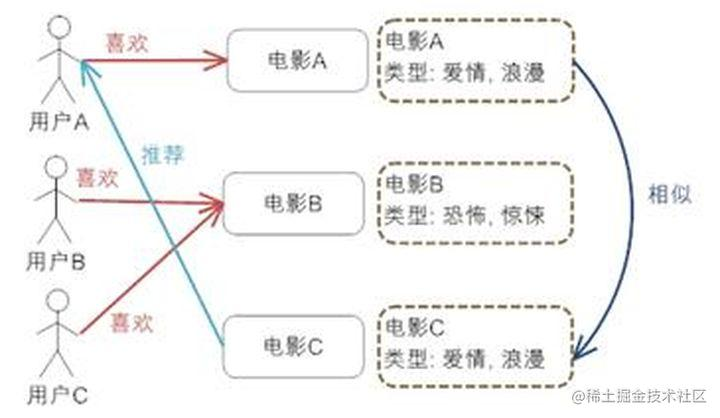#### 2.4.2 协同过滤

• User-Based CF: 要考虑的是用户和用户之间的相似度，只要找出相似用户喜欢的物品，并预测目标用户对对应物品的评分，就可以找到评分最高的若干个物品推荐给用户。
• Item-Based CF: 要考虑的是物品和物品之间的相似度，只有找到了目标用户对某些物品的评分，那么我们就可以对相似度高的类似物品进行预测，将评分最高的若干个相似物品推荐给用户。
• Model-Based CF: 目前最主流的协同过滤类型了，所含算法是非常之多的，如矩阵分解、关联算法、聚类算法、深度学习、图模型等等。

• 无需领域知识：不需要相关领域知识，因为Embedding是自动学习的。
• 发掘用户兴趣：该模型可以帮助用户发现新的兴趣点。 系统可能并不知道用户对给定的item的兴趣度，但是模型仍会推荐给他，因为相似的用户有着相同的兴趣点。
• 很好的初始模型：在某种程度上，该方法仅需要反馈矩阵即可训练矩阵分解模型。 而且该方法不需要上下文特征。 实际上，该方法可以用作多个召回队列中的一个。

• 冷启动问题：模型预测结果是给定的（用户，商品）相应Embedding的点积。因此，如果在训练数据中item从未出现过，则系统也无法计算其Embedding，也无法得到相应的预测结果。此问题通常称为冷启动问题。目前可用来解决冷启动问题的方法是：

• 利用WALS进行预测。
• 启发式生成新item的embedding
• 难以融入query/item的附加特征

#### 2.4.3 基于因子分解机（FM）模型召回

FM算法的核心在于特征组合，以此来减少人工参与特征组合工作。对于FM，其优势可分以下三点:

1. FM能处理数据高度稀疏场景，SVM则不能；
2. FM具有线性的计算复杂度，而SVM依赖于support vector。
3. FM能够在任意的实数特征向量中生效。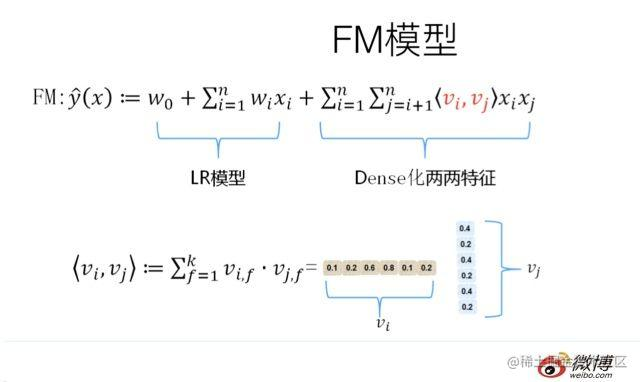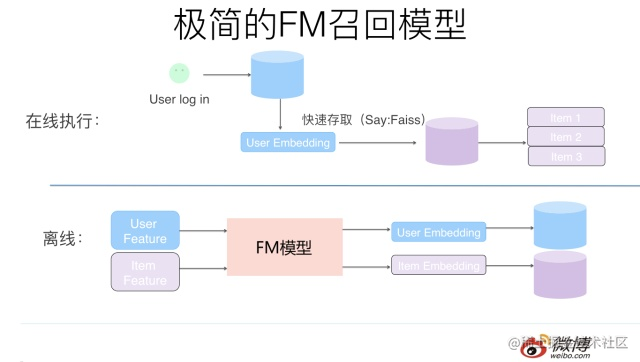#### 2.4.5 基于DNN

• 使用附加特征（即queryID /itemID以外的其他特征）困难。 因此只能对训练集中存在的用户或item进行推荐。
• 推荐的相关性。 正如前文所描述的那样，倾向于向所有人推荐热门item，尤其是在使用点积作为相似性度量时。 难以刻画特定的用户兴趣。

## 3. 排序

• 矩阵分解模型的相关item。
• 根据各类标签下的用户item。
• “本地”与“非本地”项目； 也就是说，要考虑地理信息。
• 热门或流行item。
• 社交网络； 也就是朋友喜欢或推荐的item。

### 3.1 建模目标

#### 3.1.1 pointwise、pairwise、listwise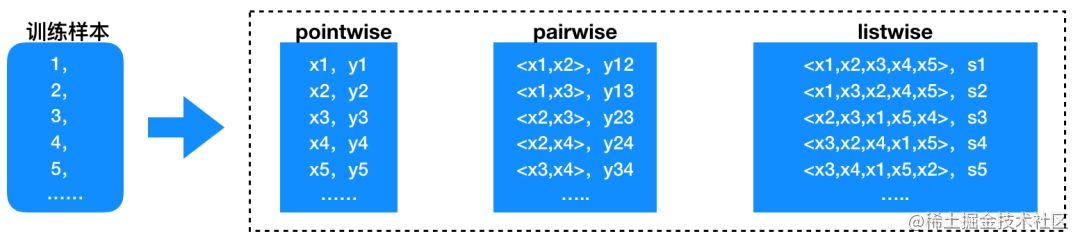### 3.3 常见模型

#### 3.3.1 LR

lr是比较简单的线性模型，通过学习用户点击行为来构建CTR预估。利用logistic回归构建推荐算法模型，具体模型如下面公式：

$\log \left ( \frac{p}{1-p} \right )=\sum_{n}^{i=1}w_{i}F_{i}$

#### 3.3.2 LR + GBDT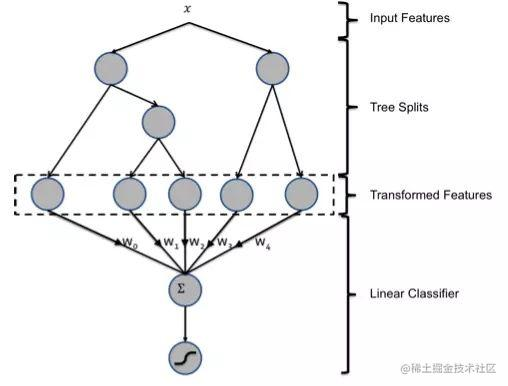#### 3.3.3 Wide&Deep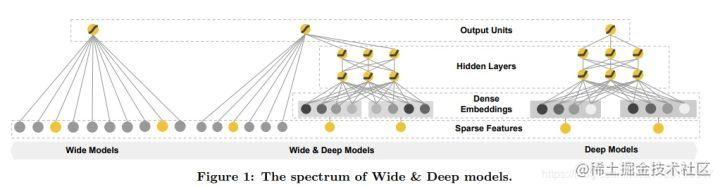#### 3.3.4 DeepFM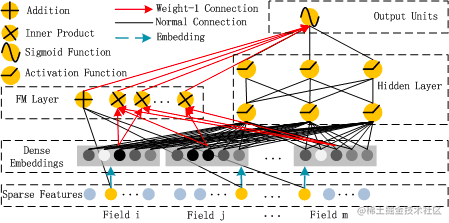DeepFM 的结构其实很简单：采取Wide & Deep的框架，差异在于将Wide部分的LR替换为了FM，从而自动构造二阶特征叉乘，而非手工设计叉乘。其中，DeepFM的输入可由连续型变量和类别型变量共同组成，且类别型变量需要进行One-Hot编码。而正由于One-Hot编码，导致了输入特征变得高维且稀疏。应对的措施是：针对高维稀疏的输入特征，采用word2vec的词嵌入方式，把高维稀疏的向量映射到相对低维且向量元素都不为零的空间向量中。由上面网络结构图可以看到，DeepFM 包括 FM和 DNN两部分，所以模型最终的输出也由这两部分组成：

$\hat{y}=sigmoid(y_{FM}+y_{DNN})$

### 3.4 模型排序效果评估

1. (深入理解推荐系统：召回)[zhuanlan.zhihu.com/p/115690499].

2. (深入理解推荐系统：排序)[zhuanlan.zhihu.com/p/138235048].

3. (推荐系统概述)[github.com/datawhalech…]